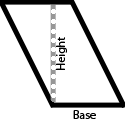## Area of a Parallelogram

### Area: Learn

Area is the space within a shape. The units of area are the squares of the units of length (that is, if a shape's sides are measured in meters, then the shape's area is measured in square meters).

For a parallelogram, simply multiply the base times the height to find the area. One of the side lengths is typically used as the base. The height is then determined by a line which is drawn from the base straight up to the opposite side. The diagram below shows this.For example, a parallelogram with a base of 4 cm and a height of 3 cm has an area of 4*3 = 12 square centimeters.

### Area: Practice

00:00

What is the area of a parallelogram whose
base is cm
and
height is cm?

Press the Start Button To Begin

You have 0 correct and 0 incorrect.

This is 0 percent correct.

### Play

Game Name Description Best Score
How many correct answers can you get in 60 seconds? 0
Extra time is awarded for each correct answer.
Play longer by getting more correct.
0
How fast can you get 20 more correct answers than wrong answers? 999